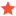Get normal with DXEngine raycastjanovromJunior MemberPosts: 39 Threads: 18 Joined: Aug 2019 Reputation: 0 10-09-2020, 11:23 AM (This post was last modified: 10-09-2020, 12:47 PM by janovrom.) Hi, I'd like to ask how can I get a normal of hit point using DXEngine raycast. I can obtain the hit result by using: DXRayHitTestResult rayHitResult = DXScene.GetClosestHitObject(pickRay, rootNode); It is possible to get rayHitResult.HitPosition and rayHitResult.TriangleIndex. But to what does the rayHitResult.TriangleIndex point to? MeshBase that can be accessed from rayHitResult.HitSceneNode does only have a buffer. I can cast it to SimpleMesh (or any other that I will use). Here it is possible to access IndexBufferArray. The issue I have is that the TriangleIndex is not divisible by 3 (I expect that the IndexBufferArray is a triangle soup). So it can't be start index of the triangle. Maybe it's triangle strip? TLDR: How can I get a normal from DXRayHitTestResult? Attached code is based on assumptions made above. Best regards, Janovsky Roman Code:```MeshBase mesh = (rayHitResult.HitSceneNode as MeshObjectNode).Mesh; int t0 = rayHitResult.TriangleIndex; int t1 = rayHitResult.TriangleIndex + 1; int t2 = rayHitResult.TriangleIndex + 2; if (mesh is SimpleMesh smPN) {    int i0 = smPN.IndexBufferArray[t0];    int i1 = smPN.IndexBufferArray[t1];    int i2 = smPN.IndexBufferArray[t2];    var p0 = smPN.VertexBufferArray[i0].Position;    var p1 = smPN.VertexBufferArray[i1].Position;    var p2 = smPN.VertexBufferArray[i2].Position;    // Compute the normal (we want to stick to the plane that is the triangle)    var e1 = p1 - p0;    var e2 = p2 - p0;    var n = Vector3.Cross(e1, e2);    n.Normalize();    forward = n; }``` Edit: If the VertexBufferArray is accessed using directly t0, t1, and t2, it produces correct results half of a time, but can be outside of array range. Find ReplyabenedikAdministratorPosts: 641 Threads: 8 Joined: Sep 2009 Reputation: 10 10-12-2020, 08:44 AM TriangleIndex is an index of a triangle and not an index of a TriangleIndice. You can get the index in the TriangleIndices collection or in IndexBuffer by multiplying TriangleIndex by 3 - for example, triangle indices for the 3rd triangle (in this case TriangleIndex is 2) start at TriangleIndice with index 6. You should change your calculation of t0 ... t2 to: PHP Code:`int t0 = rayHitResult.TriangleIndex * 3;int t1 = t0 + 1;int t2 = t0 + 2; ` Andrej Benedik Find Reply

Forum Jump:

 Users browsing this thread: 1 Guest(s)